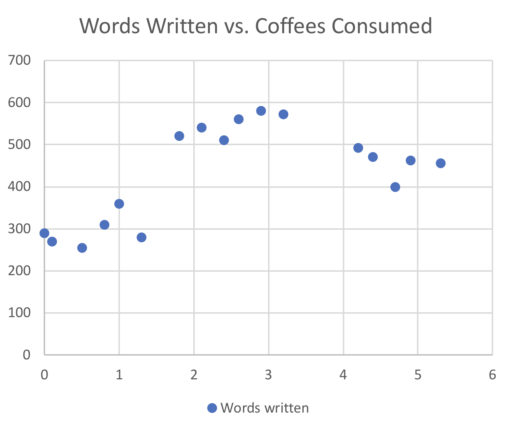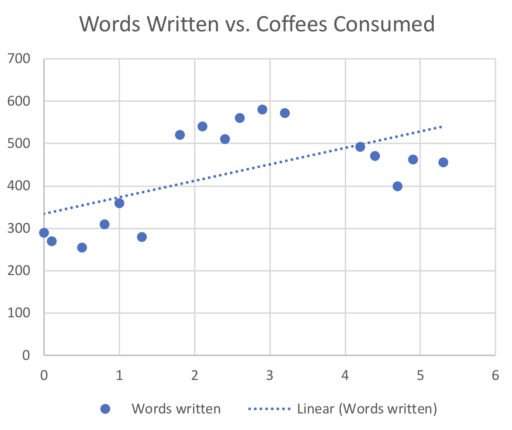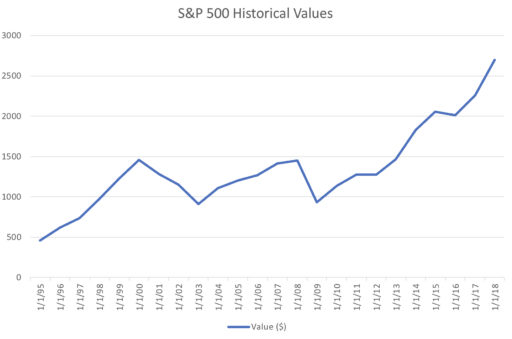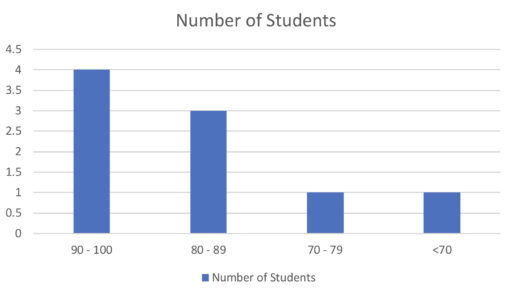Often, you want to use data and graphs to figure out what the relation between two variables is. For example, you may wonder whether your scores improve as you spend more time studying. Or you may wonder how years of education affect one’s lifetime earnings. Scatterplots allow you to plot one variable against another in order to determine what the relationship between them is.

Here’s an example of a scatterplot:Here, we can see how consuming coffees (on the x-axis, i.e. the bottom axis) affects the number of words one writes (on the y-axis, i.e. the axis on the left-hand side). (You may wonder, sensibly enough, how one can consume non-integer quantities of coffee. We presume that means people drank a partial cup of coffee).

(Part of) the data table corresponding to this graph looks like:In the left-hand column, we have the variable for the x-axis (namely the number of coffees one drank)  and on the right-hand side, we have the variable for the y-axis (the number of words one writes).

Now, you may be asked to interpret the graph above. So, for example, you may get something like:

Example 1

The above graph comes from a study of how coffee affects literary output. The researchers asked 17 people to drink as much coffee they like and recorded how many words they wrote in the next hour. How many people drank 1 or fewer cups of coffee?

Example 2

Of the people who drank two or more cups of coffee, how many wrote more than 500 words?

Sometimes, you will see a scatterplot that also has a “trend line” like so:The trend line is an attempt to infer, from the available data, what the general pattern looks like. Generally, it will be a straight line chosen (by some algorithm) to be an optimal fit for the data.

Example 3

According to the trend line in our graph, approximately how many words will someone who drank 2 cups of coffee write?

Finally, scatterplots will often use time as the variable on the x-axis. This is because we are often interested in knowing how some variable (e.g. value of a share, net worth, world record for running a marathon) changes with time. We say that time plots are the scatterplots that use time as a variable. Here is an example:The S&P 500 is an index of, roughly speaking, the share value of the 500 largest publicly traded companies. Here, we can see that it has grown considerably over the past 20 or so years.

Example 4

By (approximately) how much has the S&P 500 increased from 1/1/1999 to 1/1/12?

Example 5

By (approximately) what percentage has the S&P 500 increased from 1/1/95 to 1/1/18?

Univariate vs. Bivariate

Some graphs only use one variable. Those graphs are called univariate. Other graphs use two variables; they are called bivariate.

How can a graph use only one variable? Well consider the histogram. It tells you how many observations fall into certain brackets. So, for example:tells you that 4 students scored between 90 and 100; 3 between 80 and 89; and so on. The raw data for this kind of graph just looks like:Where we just record different observations of a single variable. Thus, we can call such graphs univariate. Other examples of univariate graphs include circle graphs and bar graphs.

By contrast, scatterplots involve two variables. See, for example:Whose data look like:Thus we see that for scatterplots, we need two variables, one for the x-axis and another for the y-axis. Thus, we call these bivariate. And since time plots are just a special kind of scatterplot (namely one that uses time as a variable), we get that time plots are also bivariate.